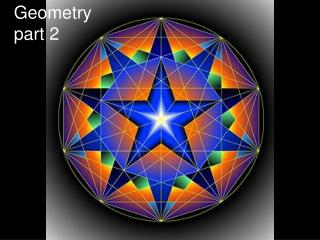DownloadDownload PresentationGeometry part 2

# Geometry part 2

Download Presentation## Geometry part 2

- - - - - - - - - - - - - - - - - - - - - - - - - - - E N D - - - - - - - - - - - - - - - - - - - - - - - - - - -
##### Presentation Transcript

1. Geometrypart 2

2. Geometric Applications are limitless….

3. 3600 There are 3600 in every circle. This initial definition allows for further angles to be identified

4. 1800 900 450 These are some more common angles

5. Supplementary Angles (SAT) Supplementary Angles must add to 180O A + B = 180O A B

6. Complementary Angles (CAT) Complementary Angles must add to 90O A + B = 90O A B

7. Opposite Angle Theorem (OAT) If 2 lines intersect, then the opposite angles are equal. O X X O

8. Shape Theorems

9. Sum of the Angles of a Triangle Theorem (SATT) The sum of the Interior Angles of a Triangle is 180O a + b + c = 180O a b c

10. Isosceles Triangle Theorem(ITT) The base angles of an Isosceles Triangle are equal X Y X

11. Equilateral Triangle Theorem(ETT) X X = 60O X X

12. Stop here and complete the worksheet

13. Exterior Interior (EI) An exterior angle equals the sum of the opposite two interior angles. D = A + B A B D

14. Exterior Angles (EA) The exterior angles of a triangle and a quadrilateral sum to 360O A A + B + C = 360O B C

15. Sum of the Angles Of a Quadrilateral Theorem (SAQT) The sum of the Interior Angles of a Quadrilateral is 360O a + b + c + d = 360O a d b c

16. Exterior Angles The exterior angles of a triangle and a quadrilateral sum to 360O A + B + C + D = 360O D A C B

17. From the green workbook Page 214 Page 217 Page 220 Page 224

18. HomeFun YA!!! Page 348 1-4 Page 352 1b, c, d, f, h Page 365 1a,c 2a,b